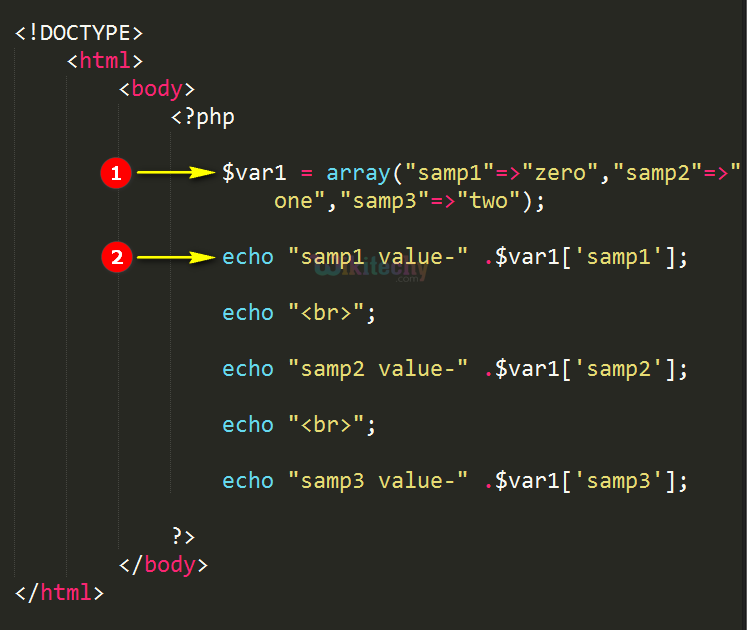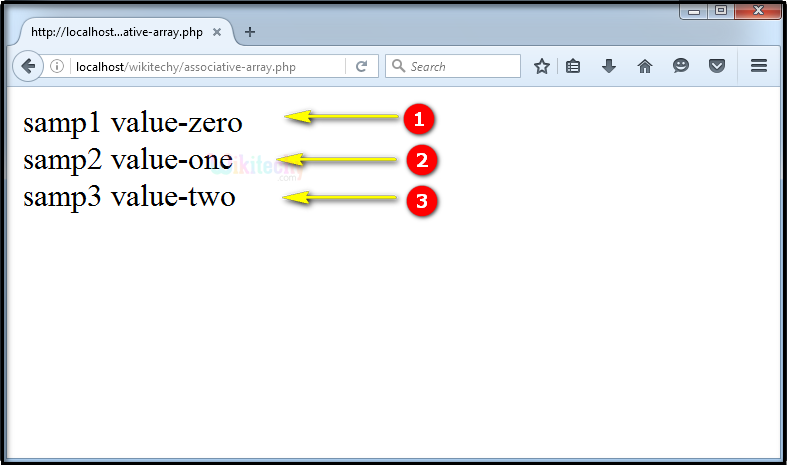# php tutorial - PHP Associative Arrays - php programming - learn php - php code - php script

• In php the array index starts from zero (0).
• But in terms of associative array, it supports index value with string data.So, the user can define the index.

## Advantage of using Associative Arrays

• We can use the associative array like a list, dictionary, collection, stack, queue, etc.…
• We can create different types of sorting process using Associative Array.
• In php we can merge the two associative arrays using php functions.

## php examples Code Explanation :1. In this statement we define the associative array for the variable “\$var1” by using array function and its value is declared by “key” in which, more than one values are separated by using commas (,) like “\$var1=array (“samp1” =>” zero”, “samp2” =>”one”, “samp3” =>” two”) and finally the declaration end with semicolon (;).
2. Echo statement echo "samp1 value-" .\$var1['samp1']; will print the key with its string value. Same thing applies for the other two echo statements.

## php tutorials Sample Output :1. Here in this output, for the key “samp 1” with its value “zero” has been printed in the output as “samp1 value-zero”.
2. Here in this output, for the key “samp 2” with its value “one” has been printed in the output as “samp2 value-one”.
3. Here in this output, for the key “samp 3” with its value “two” has been printed in the output as “samp3 value-two”.